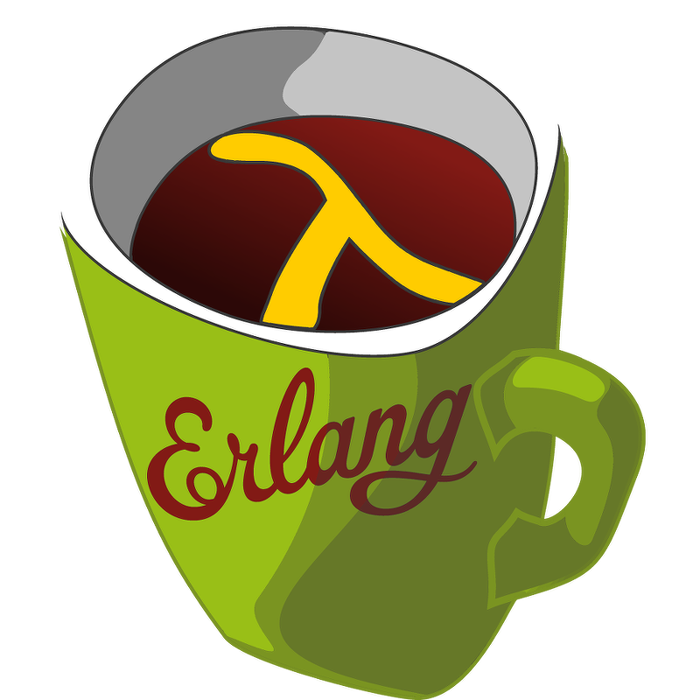This week's LFE Friday was translated with permission from the Erlang Thursday series by Steven Proctor. This week's translator: Robert Virding.

For today's LFE Friday we continue looking at the `queue` module and look at queue:peek/1 from the Extended API.

`queue:peek/1` takes a queue as it's argument and returns either the atom `empty` if the queue is empty, or `#(value item)` where `item` is the item at the head of the queue.

``````> (set queue-one (queue:from_list '(1 2 3 4 5)))
#((5 4) (1 2 3))
> (queue:peek queue-one)
#(value 1)
> (set empty-queue (queue:new))
#(() ())
> (queue:peek empty-queue)
empty``````

`queue:peek/1` does not modify the existing queue at all either, so we can call it once as seen above, or multiple times as below, and the queue we peeked at doesn't change.

``````> (set queue-two (queue:from_list '(a b c d e f)))
#((f e) (a b c d))
> (queue:peek queue-two)
#(value a)
> (queue:peek queue-two)
#(value a)
> (queue:peek queue-two)
#(value a)
> queue-two
#((f e) (a b c d))``````

And unlike we saw in the previous LFE Friday on queue:head/1, we can safely peek at an empty queue instead of getting an exception.

``````> (queue:head empty-queue)
exception error: empty
in (: queue head #(() ()))

> (queue:peek empty-queue)
empty``````

Erlang's `queue` module also contains queue:peek_r/1 which will peek at the element at the rear of the queue.

``````> (queue:peek_r empty-queue)
empty
> (queue:peek_r queue-one)
#(value 5)
> (queue:peek_r queue-one)
#(value 5)
> (queue:peek_r queue-one)
#(value 5)
> (queue:peek_r queue-two)
#(value f)
> queue-two
#((f e) (a b c d))
> queue-one
#((5 4) (1 2 3))
> empty-queue
#(() ())``````

-Proctor, Robert

14 June 2015

tutorials